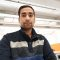Home » Core Java » Basic Operators: ^ in JavaI am Yusuf Ansari, I earned my degree in Information Technology from Guru Gobind Singh Indraprastha University. I have over two years of professional experience in Java based application development.I have worked as a backend developer mostly but also worked on the front-end part in some React.js based projects.

# Basic Operators: ^ in Java

In this post, we feature a comprehensive article about the Bitwise Operator ^ in Java.

## 1. Introduction

Bitwise XOR (exclusive or) is one of the bitwise operators which operate on individual bits. It is denoted by `^` symbol. `^` requires two arguments in order to work and it performs XOR operation between them in their binary form. XOR returns true only if both the binary arguments have different values otherwise it return false.

## 2. Usage of the bitwise operator ^ in Java

Let’s check an example using `^` operator.BitwiseXORExample.java
 01020304050607080910111213141516171819202122232425262728 `public` `class` `BitwiseXORExample {``    ``public` `static` `void` `main(String[] args) {``        ``// XOR with boolean values``        ``System.out.println(``"XOR with boolean values : "``);``        ``printValues(``true``, ``true``);``        ``printValues(``true``, ``false``);``        ``printValues(``false``, ``true``);``        ``printValues(``false``, ``false``);``        ``// XOR with int values``        ``System.out.println(``"\nXOR with int values : "``);``        ``printValues(``10``, ``11``);``        ``printValues(``11``, ``10``);``        ``printValues(``10``, ``10``);``        ``printValues(``11``, ``11``);``    ``}``    ``public` `static` `void` `printValues(``boolean` `a, ``boolean` `b) {``        ``System.out.println(``"a = "` `+ a + ``",\tb = "` `+ b + ``",\ta^b = "` `+ (a ^ b));``    ``}``    ``public` `static` `void` `printValues(``int` `a, ``int` `b) {``        ``int` `c = (a ^ b);``        ``System.out.println(``"a = "` `+ a + ``" (binary form : "` `+ Integer.toBinaryString(a) + ``") \tb = "` `+ b``                ``+ ``" (binary form : "` `+ Integer.toBinaryString(b) + ``"),\ta^b = "` `+ c + ``" (binary form : "``                ``+ Integer.toBinaryString(c) + ``")"``);``    ``}``}`
Output
 0102030405060708091011 `XOR with boolean values : ``a = ``true``,   b = ``true``,   a^b = ``false``a = ``true``,   b = ``false``,  a^b = ``true``a = ``false``,  b = ``true``,   a^b = ``true``a = ``false``,  b = ``false``,  a^b = ``false``XOR with int values : ``a = 10 (binary form : 1010)     b = 11 (binary form : 1011),    a^b = 1 (binary form : 1)``a = 11 (binary form : 1011)     b = 10 (binary form : 1010),    a^b = 1 (binary form : 1)``a = 10 (binary form : 1010)     b = 10 (binary form : 1010),    a^b = 0 (binary form : 0)``a = 11 (binary form : 1011)     b = 11 (binary form : 1011),    a^b = 0 (binary form : 0)`
Here we can see, for boolean values, `^` operator return false if both input variables `a` and `b` have same values, else it returns true.However, when we use `^` operator with integer values, XOR operation is performed in their binary form on each bit. For example when `a` and `b` has value 10 and 11, and their binary representation is 1010 and 1011 respectively, then `a^b` is performed as follows:0 `^` 1=11 `^` 1=00 `^` 0=01 `^` 1=0Another usage of the bitwise operator is condition check.Let’s consider a problem where we have to find those numbers which are divisible by either 5 or 3, but not both.BitWiseXORForConditionCheck.java
 01020304050607080910111213141516171819202122232425 `public` `class` `BitWiseXORForConditionCheck {``    ``public` `static` `void` `main(String[] args) {``        ``int` `rangeStart = ``5``;``        ``int` `rangeEnd = ``20``;``        ``System.out.println(``"old way : "``);``        ``for` `(``int` `i = rangeStart; i <= rangeEnd; i++) {``            ``if` `(isDivisbleByEither3Or5OldWay(i))``                ``System.out.print(i + ``"\t"``);``        ``}``        ``System.out.println(``"\nXOR way :"``);``        ``for` `(``int` `i = rangeStart; i <= rangeEnd; i++) {``            ``if` `(isDivisbleByEither3Or5(i))``                ``System.out.print(i + ``"\t"``);``        ``}``    ``}``    ``static` `boolean` `isDivisbleByEither3Or5(``int` `a) {``        ``return` `(a % ``3` `== ``0``) ^ (a % ``5` `== ``0``);``    ``}``    ``static` `boolean` `isDivisbleByEither3Or5OldWay(``int` `a) {``        ``return` `((a % ``3` `== ``0``) && !(a % ``5` `== ``0``)) || (!(a % ``3` `== ``0``) && (a % ``5` `== ``0``));``    ``}``}`
Output
 1234 `old way : ``5   6   9   10  12  18  20  ``XOR way :``5   6   9   10  12  18  20`
Here as we can see, the method which uses `^` looks cleaner and readable than the traditional approach using `&&` operator.

# Do you want to know how to develop your skillset to become a Java Rockstar?

## Subscribe to our newsletter to start Rocking right now!

### and many more ....